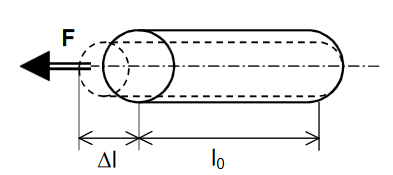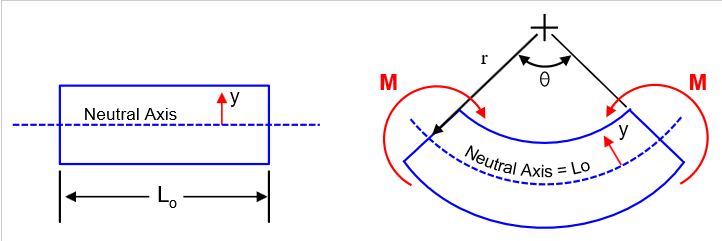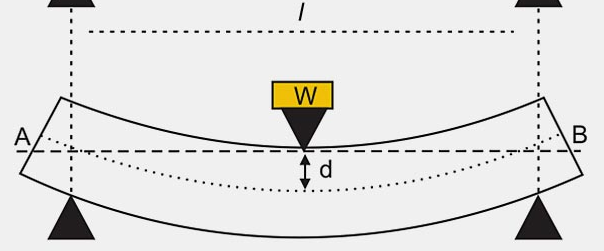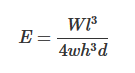The modulus of elasticity (= Young’s modulus) E is  a material property, that describes its stiffness a nd  is therefore one of the most important properties o f solid materials. When  a  material  deforms  elastically,  the  amount  of  deformation  likewise  depends  on  the  size  of  the  material, but the strain for a given stress is alwa ys the same and the two are related by Hooke ́s Law  (stress is directly proportional to strain):

σ = E × ε

σ is stress
E is modulus of elasticity
ε is strain (unitless)

Elasticity module is calculated as follows; dividing stress by strain.

E = σ / ε

What is strain in Tension?

Strain in tension is defined as the change of the length divided by the original (initial) lengthε = ( Δl ) / lo (strain under tension)

Δl = Change in Length
l= Original Length

How about what is the strain in Flexural stress?

When the material is under bending load, strain is calculated as dividing the distance from the neutral axis by the curve radius.ε = y / r (strain under bending)

How to Calculate Modules of Elasticity?E = Modules of Elasticity (Young's Modules)